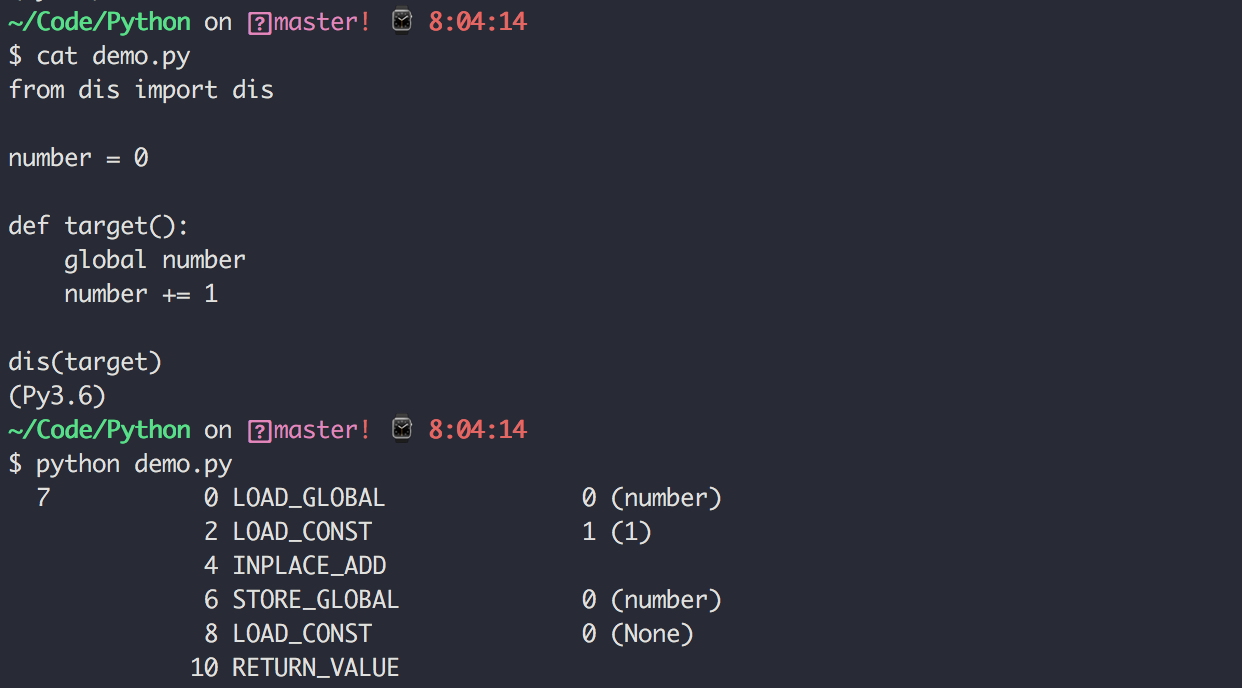# 零基础学 Python（86）：浅谈线程安全那些事儿

## 1. 线程不安全是怎样的？

from threading import Thread, Lock

number = 0

def target():
global number
for _ in range(1000000):
number += 1

print(number)


1459782
1379891
1432921


## 2. 什么是原子操作？

Python官方文档上，列出了一些常见原子操作

L.append(x)
L1.extend(L2)
x = L[i]
x = L.pop()
L1[i:j] = L2
L.sort()
x = y
x.field = y
D[x] = y
D1.update(D2)
D.keys()


i = i+1
L.append(L[-1])
L[i] = L[j]
D[x] = D[x] + 1• BINARY_ADD ：将两个值相加
• STORE_GLOBAL： 将相加后的值重新赋值• LOAD_GLOBAL：加载全局变量
• LOAD_ATTR： 加载属性，获取 update 方法
• LOAD_FAST：加载 new 变量
• CALL_FUNCTION：调用函数
• POP_TOP：执行更新操作

## 3. 实现人工原子操作

from threading import Thread, Lock

number = 0
lock = Lock()

def target():
global number
for _ in range(1000000):
with lock:
number += 1

print(number)


1. Event
2. Condition
3. Queue

## 参考文章：

https://zhuanlan.zhihu.com/p/34150765

https://juejin.im/post/5b129a1be51d45068a6c91d4#comment